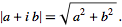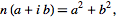# Differences between Gaussian integers with norm 25

• A
• Ventrella

#### Ventrella

I am exploring Gaussian integers in terms of roots, powers, primes, and composites. I understand that multiplying two integers with norm 5 result in an integer with norm 25. I get the impression that there are twelve unique integers with norm 25, and they come in two flavors:

(1) Four of them are 0+5i, 0-5i, and 5+0i, -5+0i. They lie on the orthogonal (real and imaginary) axes.

(2) The other eight are 3+4i, 3-4i, -3+4i, -3-4i, 4+3i, 4-3i, -4+3i and -4-3i. These do not lie on the orthogonal axes.

Each of these twelve integers is the product of two integers with norm 5, but it matters which ones.

If there are any insights as to these kinds of Gaussian integers (having different symmetries but the same norm), I would appreciate any thoughts. Also, I assume these become more numerous as norms get larger?

Thanks!

-Jeffrey

The Gaussian integers you list have norm 5, not 25. The norm of a complex number is the square root of the sum of squares of the real and complex components. More here.

For any positive integer n there will be at least four Gaussian integers with that norm: ##n\,i,-n\,i,n,-n##. There will be additional Gaussian integers with that norm iff n is the largest number in a Pythagorean Triple. Where that is the case, say ##n^2 = m^2 + k^2##, there are eight additional Gaussian integers with norm n, being ##\pm m\pm k\,i## and ##\pm k\pm m\,i##.

So studying Gaussian integers with the property you seek is equivalent to studying Pythagorean Triples, on which there has been plenty of work. The linked wiki article on Pythagorean Triples has a section dedicated to their relationship to Gaussian integers here. The article proves that there are infinitely many Pythagorean Triples, and hence infinitely many integers that have at least twelve Gaussian integers with that norm. I think, based on the following statement in the wiki under heading 'General Properties', that it is possible for an integer to be the largest number in more than one Pythagorean triple, in which case there would be at least 4 + 2 x 8 = 20 Gaussian integers with that norm:

wiki said:
There are no Pythagorean triples in which the hypotenuse and one leg are the legs of another Pythagorean triple

Hi andrewkirk,

According to Wikipedia,

"The norm of a Gaussian integer is ... the square of its absolute value as a complex number ... a sum of two squares." I believe you are referring to the "Euclidean norm", which I understand to be the same as the complex modulus, or the absolute value.

According to Wolfram... "One definition of the norm of a Gaussian integer is its complex modulusAnother common definition (e.g., Herstein 1975; Hardy and Wright 1979, p. 182; Artin 1991; Dummit and Foote 2004) defines the norm of a Gaussian integer to bethe square of the above quantity.

My use of the term is also consistent with this list: https://en.wikipedia.org/wiki/Table_of_Gaussian_integer_factorizations

I read somewhere that there is confusion as to the actual definition. I would love to find a good name for the norm I am referring to.

Anyway...thanks for the reference to Pythagorean Triples - that is very interesting. I will explore this further. I think that should help me get a grasp of these kinds of Gaussian integers.

Thanks :)
-j

The Gaussian integers you list have norm 5, not 25. The norm of a complex number is the square root of the sum of squares of the real and complex components. More here.

For any positive integer n there will be at least four Gaussian integers with that norm: ##n\,i,-n\,i,n,-n##. There will be additional Gaussian integers with that norm iff n is the largest number in a Pythagorean Triple. Where that is the case, say ##n^2 = m^2 + k^2##, there are eight additional Gaussian integers with norm n, being ##\pm m\pm k\,i## and ##\pm k\pm m\,i##.

So studying Gaussian integers with the property you seek is equivalent to studying Pythagorean Triples, on which there has been plenty of work. The linked wiki article on Pythagorean Triples has a section dedicated to their relationship to Gaussian integers here. The article proves that there are infinitely many Pythagorean Triples, and hence infinitely many integers that have at least twelve Gaussian integers with that norm. I think, based on the following statement in the wiki under heading 'General Properties', that it is possible for an integer to be the largest number in more than one Pythagorean triple, in which case there would be at least 4 + 2 x 8 = 20 Gaussian integers with that norm: